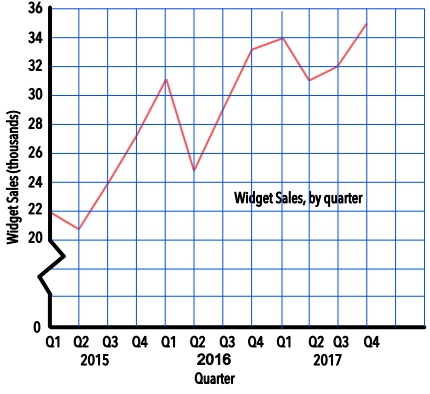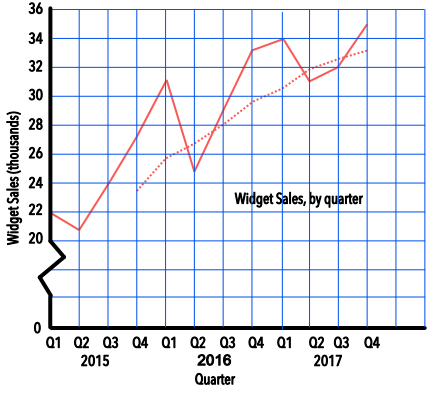Time Series Data

## Time Series Data

A graph that shows Time Series Data is a plot of value against time, with time shown along the bottom of the graph.

More than one set of data may be plotted on a graph: for example, plotting a comparison of rainfall between two different places.

Time series data can be used to determine a Moving Average that smooths out the peaks and troughs of a graph so that any trend, or movement, can be determined. A moving average uses the mean of the number of periods: for example, a three-month moving average will add the values for three consecutive months and divide by 3. A moving average can only be plotted when the number of periods in the moving averages are available.

## Example 1

The sales of widgets are noted on a table for each Quarter over the previous three years. Plot the time series on a graph.

(A quarter is a period of three months eg the first quarter in a year is January-February-March)

 Quarter 2015 Q1 2015 Q2 2015 Q3 2015 Q4 2016 Q1 2016 Q2 Widgets (thousands) 22 21 24 27 31 25 Quarter 2016 Q3 2016 Q4 2017 Q1 2017 Q2 2017 Q3 2017 Q4 Widgets (thousands) 29 33 34 31 32 35

Remember to title the graph, and to name the axes and provide scales.## Example 2

For the sales of widgets, above, plot the 4-quarter moving average on the same graph.

Moving average for 2015 Q4 = (22 + 21 + 24 + 27) ÷ 4 = 23.5

Moving average for 2016 Q1 = (21 + 24 + 27 + 31) ÷ 4 = 25.8

Moving average for 2016 Q2 = (24 + 27 + 31 + 25) ÷ 4 = 26.7 etc..

 Quarter 2015 Q1 2015 Q2 2015 Q3 2015 Q4 2016 Q1 2016 Q2 Widgets (thousands) 22 21 24 27 31 25 4Q Moving Average 23.5 25.8 26.7 Quarter 2016 Q3 2016 Q4 2017 Q1 2017 Q2 2017 Q3 2017 Q4 Widgets (thousands) 29 33 34 31 32 35 4Q Moving Average 28.0 29.5 30.3 31.8 32.5 33.0